#Function Repository Resource:

# SameInstanceQ

Determine if two Wolfram Language expressions come from the same instance

Contributed by: Jason Biggs
 ResourceFunction["SameInstanceQ"][expr1,expr2] returns True if expr1 and expr2 share the same instance. ResourceFunction["SameInstanceQ"][expr1,expr2,…] returns True if all the expri are the same instance.

## Details and Options

Two expressions share the same instance if they represent pointers to the same data—in other words, if they occupy the same location in memory.

## Examples

### Basic Examples (3)

Two expressions that are identical are considered SameQ:

 In:=Out=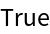However, they are different instances of the same data:

 In:=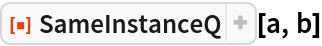Out=By contrast, the value for c in the below is literally the same value for a, since both reference the same memory location:

 In:=Out=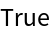### Scope (1)

SameInstanceQ can accept any number of arguments:

 In:=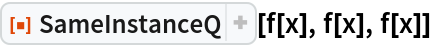Out=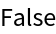In:=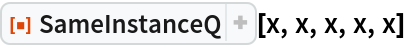Out=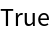### Properties and Relations (5)

SameInstanceQ always returns True for a single argument:

 In:=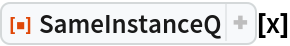Out=In:=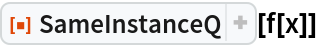Out=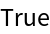Compare to SameQ:

 In:=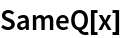Out=In:=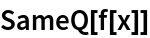Out=SameInstanceQ[] is defined to be True:

 In:=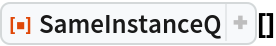Out=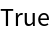This matches the behavior of SameQ:

 In:=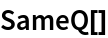Out=Memory is typically allocated for each new expression that is created, even if it shares the same value as an existing expression:

 In:=Out=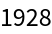In this case, a and b have identical values:

 In:=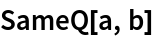Out=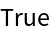However, their values are stored separately in memory, since each expression was created separately:

 In:=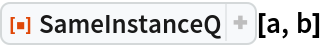Out=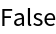Reusing expressions that are already created can save memory:

 In:=Out=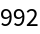The value for a is literally the same value for b, since both reference the same memory location:

 In:=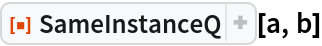Out=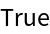Many simple expressions are already preallocated in the kernel:

 In:=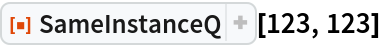Out=In:=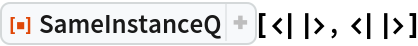Out=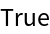These are not necessarily atomic values:

 In:=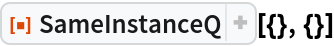Out=Atomic expressions that are SameQ are not necessarily SameInstanceQ:

 In:=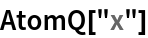Out=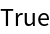In:=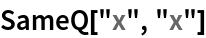Out=In:=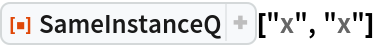Out=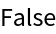### Possible Issues (2)

Having all of the corresponding parts of expressions be SameInstanceQ does not imply the parent expressions are SameInstanceQ:

 In:=In:=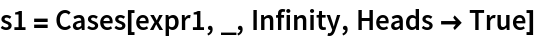Out=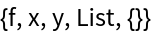In:=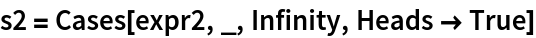Out=In:=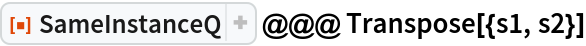Out=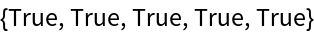In:=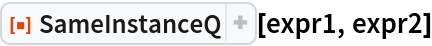Out=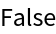Small integers are already created in memory, so they are always the same instance:

 In:=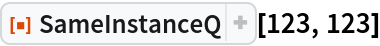Out=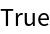Larger integers are created as needed:

 In:=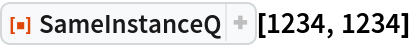Out=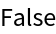Find the range of small integers that are preallocated in memory:

 In:=Out=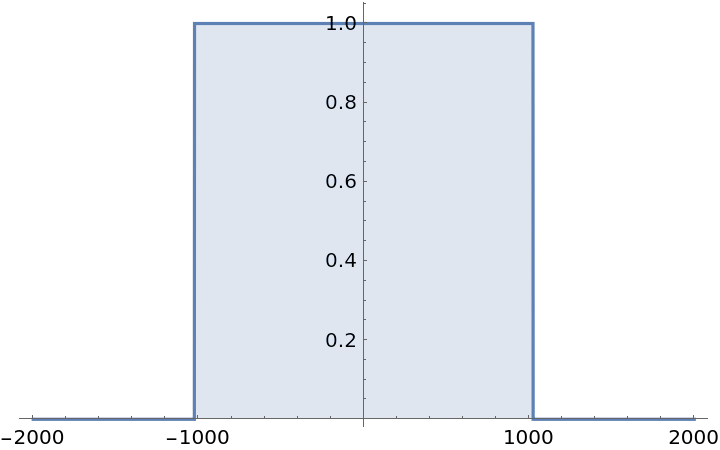### Neat Examples (1)

Test modifications on expressions to see which lead to new instances:

 In:=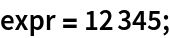In:=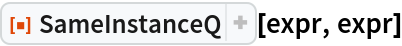Out=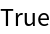In:=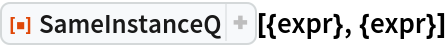Out=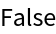In:=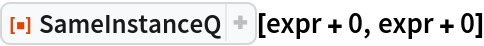Out=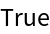In:=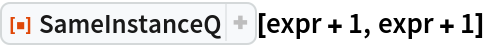Out=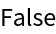In:=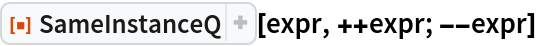Out=JasonB

## Version History

• 1.0.0 – 11 May 2020